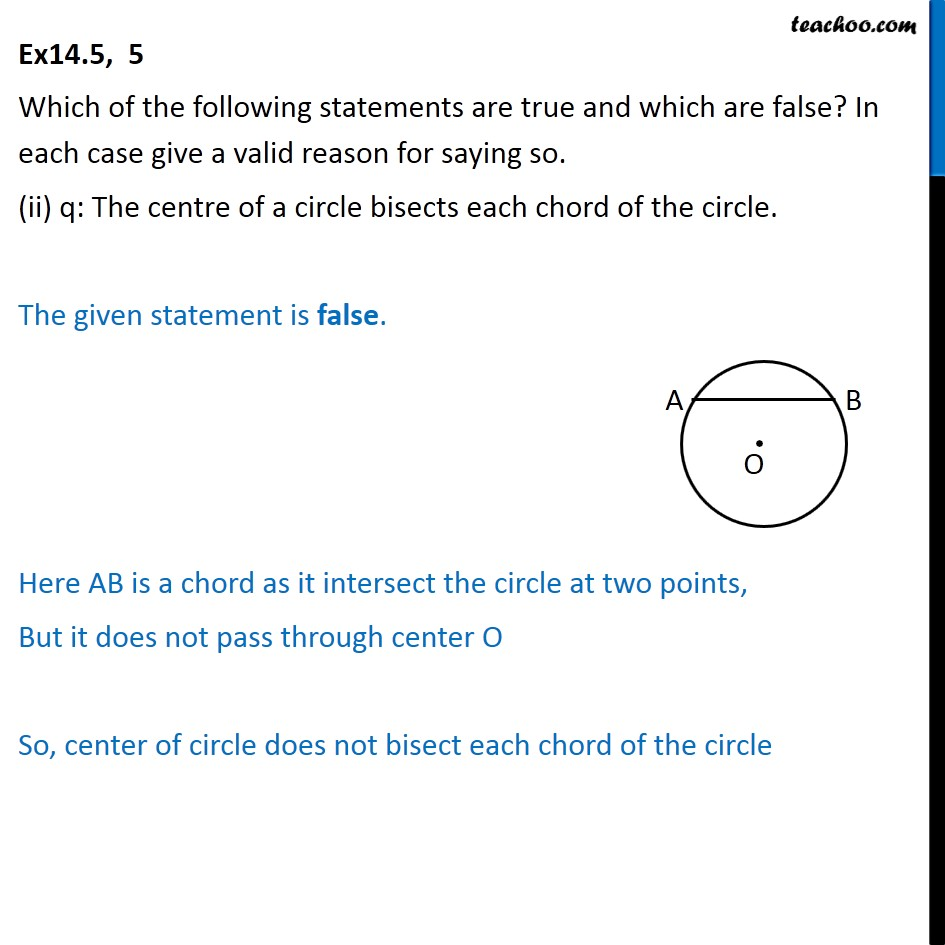1. Chapter 14 Class 11 Mathematical Reasoning (Deleted)
2. Serial order wise
3. Ex 14.5

Transcript

Ex14.5, 5 Which of the following statements are true and which are false? In each case give a valid reason for saying so. (ii) q: The centre of a circle bisects each chord of the circle. The given statement is false. Here AB is a chord as it intersect the circle at two points, But it does not pass through center O So, center of circle does not bisect each chord of the circle